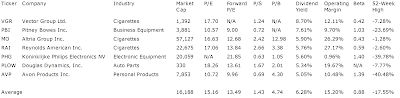## Pages

### 7 Consumer Goods High Yields

High Yields From The Consumer Goods Sector by Dividend Yield - Stock, Capital, Investment. Here is a current sheet stocks from the consumer goods sector. 15 stocks have such a high yield of which 8 have a market capitalization of less than USD 300 million. Below are the high yields above this market capitalization.

Here are the 3 top dividend stocks by market capitalization:

Vector Group (NYSE:VGR) has a market capitalization of \$1.39 billion. The company employs 512 people, generates revenues of \$1,063.29 million and has a net income of \$54.08 million. The firm’s earnings before interest, taxes, depreciation and amortization (EBITDA) amounts to \$122.10 million. Because of these figures, the EBITDA margin is 11.48 percent (operating margin 10.47 percent and the net profit margin finally 5.09 percent).

The total debt representing 58.70 percent of the company’s assets. Due to the financial situation, the return on equity amounts to percent. Finally, earnings per share amounts to \$0.99 of which \$1.47 were paid in form of dividends to shareholders last fiscal.

Here are the price ratios of the company: The P/E ratio is 17.67, Price/Sales 1.31 and Price/Book ratio is not calculable. Dividend Yield: 8.70 percent. The beta ratio is 0.42.Long-Term Stock Chart Of Vector Group Ltd. (Click to enlarge)

Pitney Bowes (NYSE:PBI) has a market capitalization of \$3.88 billion. The company employs 30,700 people, generates revenues of \$5,425.25 million and has a net income of \$328.81 million. The firm’s earnings before interest, taxes, depreciation and amortization (EBITDA) amounts to \$951.31 million. Because of these figures, the EBITDA margin is 17.53 percent (operating margin 9.85 percent and the net profit margin finally 6.06 percent).

The total debt representing 50.84 percent of the company’s assets. Due to the financial situation, the return on equity amounts to percent. Finally, earnings per share amounts to \$1.84 of which \$1.46 were paid in form of dividends to shareholders last fiscal.

Here are the price ratios of the company: The P/E ratio is 10.56, Price/Sales 0.72 and Price/Book ratio is not calculable. Dividend Yield: 7.61 percent. The beta ratio is 1.03.Long-Term Stock Chart Of Pitney Bowes Inc. (Click to enlarge)

Altria Group (NYSE:MO) has a market capitalization of \$57.13 billion. The company employs 10,000 people, generates revenues of \$24,363.00 million and has a net income of \$3,907.00 million. The firm’s earnings before interest, taxes, depreciation and amortization (EBITDA) amounts to \$6,504.00 million. Because of these figures, the EBITDA margin is 26.70 percent (operating margin 25.56 percent and the net profit margin finally 16.04 percent).

The total debt representing 32.60 percent of the company’s assets and the total debt in relation to the equity amounts to 234.86 percent. Due to the financial situation, the return on equity amounts to 84.01 percent. Finally, earnings per share amounts to \$1.67 of which \$1.46 were paid in form of dividends to shareholders last fiscal.

Here are the price ratios of the company: The P/E ratio is 16.63, Price/Sales 2.34 and Price/Book ratio 11.18. Dividend Yield: 5.90 percent. The beta ratio is 0.43.Long-Term Stock Chart Of Altria Group, Inc. (Click to enlarge)

Here is the full table with some fundamentals (TTM):7 Consumer Goods High Yields (Click to enlarge)

Take a closer look at the full table. The average price to earnings ratio (P/E ratio) amounts to 15.2 while the forward price to earnings ratio is 13.5. The dividend yield has a value of 6.3 percent. Price to book ratio is 4.7 and price to sales ratio 1.4. The operating margin amounts to 15.2 percent.

Related stock ticker symbols:
VGR, PBI, MO, RAI, PHG, PLOW, AVP

Selected Articles: